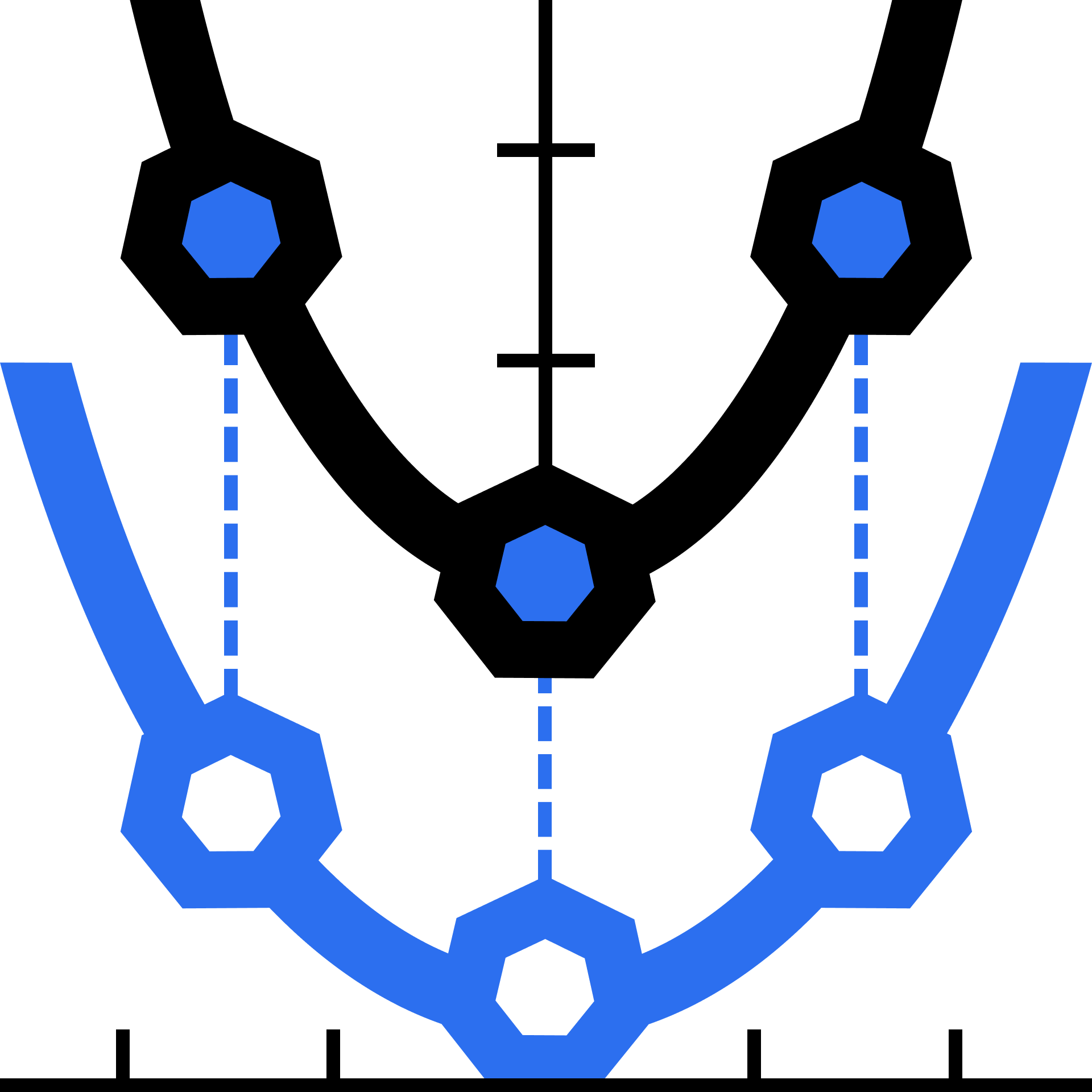Learn about functions and how to work with them. Then, take a deep dive into quadratics.

What is a Function?

Tables and Graphs

Domain and Range

Rigid Transformations

Vertical Stretch and Shrink

Horizontal Stretch and Shrink

Factored Form

Finding Roots

Intro to Complex Numbers

Intro to Polynomials

Arithmetic and Finding Roots

Symmetry and Roots in Graphs

Direct and Inverse Variation

Variation with Powers

Rational Functions and Domain

End Behavior

Problem Solving

Absolute Value

Piecewise Functions

Floor and Ceiling

Problem Solving

### Course description

Understand functions as set mappings, tables, and graphs. Using these tools, learn how to work with functions and transform them and their graphs. Then, use the framework of functions to do a deep dive on quadratics. You'll explore factoring, completing the square, learn about polynomials, and eventually develop the quadratic equation.

### Topics covered

• Absolute Value
• Asymptotes
• Domain and Range
• Exponents
• Factoring Polynomials
• Functions
• Function Notation
• Function Transformations
• Piecewise Functions
• Projectile Motion
• Rational Functions
• The Binomial Theorem